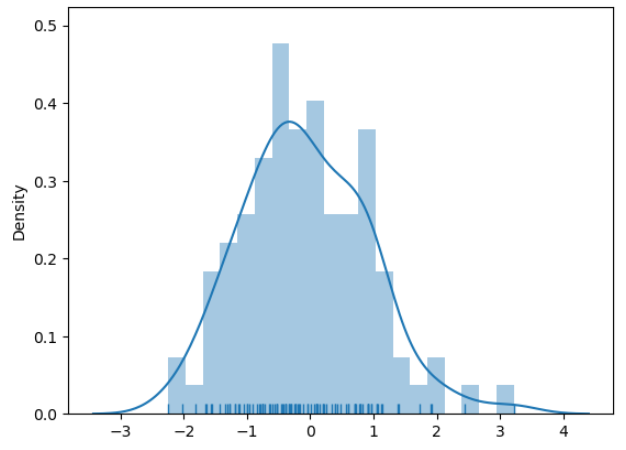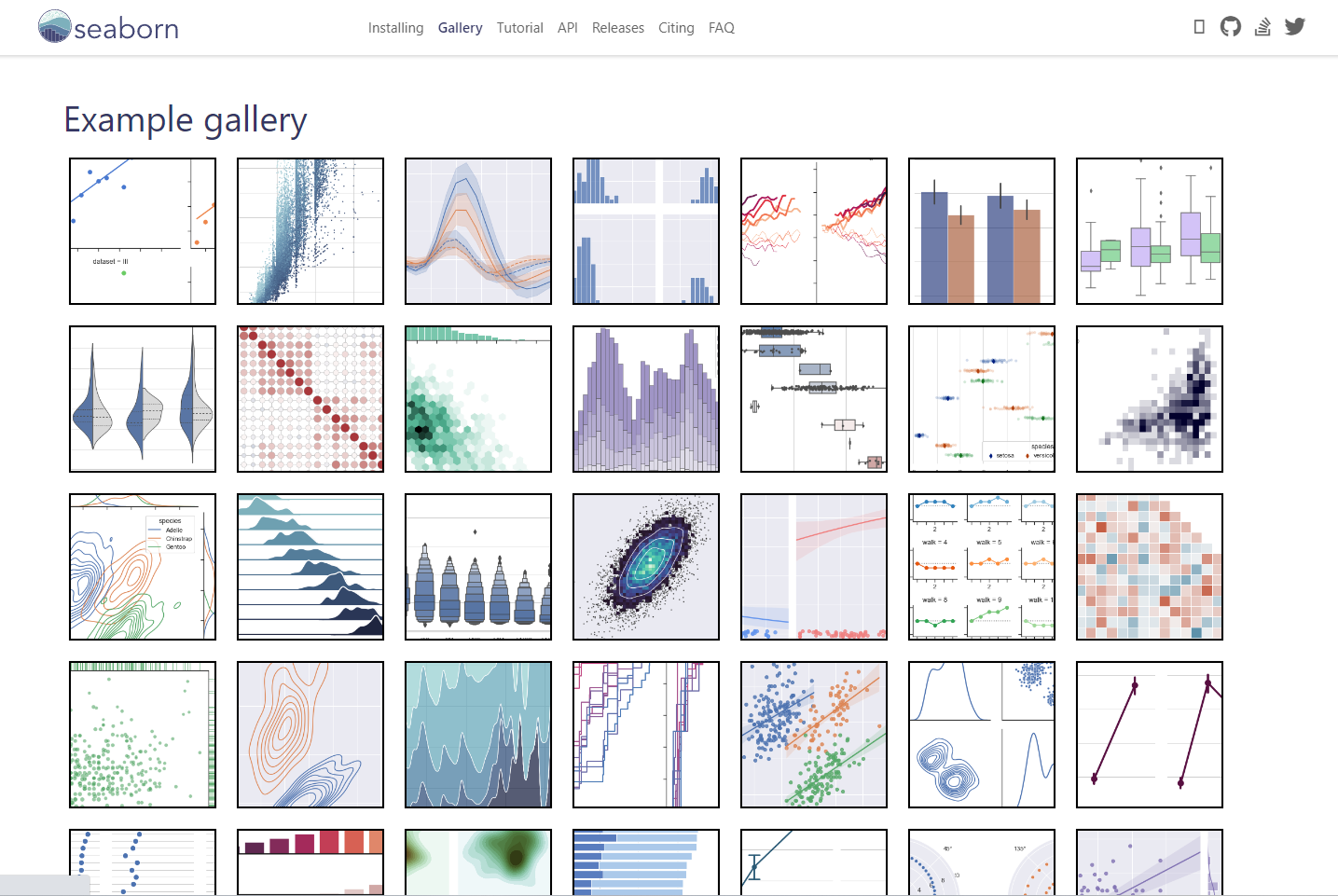LonghaoWang

V1

2023/04/01阅读：35主题：橙心

# Matplotlib vs. Seaborn? 有什么区别，用哪个？

Matplotlib是Python中最常见的库，而Seaborn是在其基础上封装的高级库。

# 两个库的介绍

Matplotlib是Python中最常见的，提供了广泛的图形选项和定制功能，可以创建复杂的、高度定制化的可视化。

Seaborn是在Matplotlib的基础上开发的高级可视化库，它专注于数据可视化的美学设计和统计图形的绘制。Seaborn更加注重对数据的探索和分析，以及对美学风格的深入研究。谢邀，Seaborn的底层是基于Matplotlib的。

Matplotlib需要大量的代码创建细节；而Seaborn是高级的封装，更为美观，但缺点是一切都是固定的：

Seaborn是用户把自己常用到的可视化绘图过程进行了函数封装，形成的一个“快捷方式”，他相比Matplotlib的好处是代码更简洁，可以用一行代码实现一个清晰好看的可视化输出。主要的缺点则是定制化能力会比较差，只能实现固化的一些可视化模板类型。

# 代码案例

## 直方图的细节

``# Importing required Libraries:import numpy as npimport pandas as pdimport matplotlib.pyplot as pltimport seaborn as sns%matplotlib inlineimport warnings # Current version of Seaborn generates a bunch of warnings that we'll ignore in this tutorialwarnings.filterwarnings("ignore")# Creating a randomly distributed dataset:x = np.random.normal(size=100)sns.distplot(x, bins=20, kde=True, rug=True)``上图看起来很怪异
seaborn的默认直方图是没有分割的，传统的Matplotlib是有分割，需要增加关键字：

``sns.distplot(x, bins=20, kde=True, rug=True, hist_kws=dict(edgecolor='k', linewidth=0.5))``
``# Loading up built-in dataset:titanic = sns.load_dataset("titanic")# Creating a Distribution plot:sns.distplot(titanic.age.dropna(), hist_kws=dict(edgecolor='k', linewidth=0.5))``
• 在上面的图中，X轴并不是从最左下角的0开始的。
• 但出于某种原因，如果我们严格希望绘图以0开头，那么它需要额外的代码行，这也是Seaborn的不方便
• 本次使用的更改轴的歧视和重点，使用的Matplotlib中的`xlim()`函数：
``plt.xlim([0, 100])sns.distplot(titanic.age.dropna(), hist_kws=dict(edgecolor='k', linewidth=0.5))``
• Matplotlib的`xlim()`通过的列表定义了X轴的下限和上限。
• 如果需要，同样，我们也可以通过添加另一行代码来设置Y轴的限制：`plt.ylim（[lower，upper]）`

## 绘图代码对比

• 上述的图绘都是用Seaborn的代码，基本控制在1-2行之内，如果引入Matplotlib，开始变得复杂：
``# Creating random datapoints:x = [1,2,3,4,5,6,7,8,9,10]y = [3,5,7,10,11,12,14,15,19,25] # Have tried to imbalance the firfit = np.polyfit(x,y,1)# fit_fn is now a function which takes in x and returns an estimate for yfit_fn = np.poly1d(fit)plt.plot(x,y, 'yo', x, fit_fn(x), '--', color='C0')plt.xlabel("Values of x")plt.ylabel("Values of y")plt.xlim(0, 11)plt.ylim(0, 30)``

``tips = sns.load_dataset("tips")sns.regplot(x="total_bill", y="tip", data=tips)``
• 两个代码绘制了完全同类的图形，但不同的是Seaborn使用单行代码就可以了。
• 继续展示Seabron和Matplotlib绘制相同图的差异：
``# Generate Data:nobs, nvars = 100, 5data = np.random.random((nobs, nvars))columns = ['Variable {}'.format(i) for i in range(1, nvars + 1)]df = pd.DataFrame(data, columns=columns)# Making a simple pairplot using Seaborn:sns.pairplot(df)``
``# Generatig Data:nobs, nvars = 100, 5data = np.random.random((nobs, nvars))columns = ['Variable {}'.format(i) for i in range(1, nvars + 1)]# Making a pairplot:fig, axes = plt.subplots(ncols=nvars, nrows=nvars, sharex='col', sharey='row')for (i, j), ax in np.ndenumerate(axes):    if i == j:        ax.annotate(columns[i], (0.5, 0.5), xycoords='axes fraction',                    ha='center', va='center', size='large')    else:        ax.scatter(data[:,i], data[:,j], color='lightblue')    ax.locator_params(nbins=6, prune='both')plt.show()``
• 我们可以在上面的两个图中观察到相当大的差异。即使在Matplotlib代码中有很大定制绘图细节的可能，但这需要更多的代码行或参数。
• 也许我们更需要Seaborn的灵活性，使我们能够以最少的代码行数实现同样的目标，而这种统计表示效率是科研中最重要的。

# 学习方法

• Seaborn官方文档：https://seaborn.pydata.org/documentation.html
• Coursera的数据可视化课程：https://www.coursera.org/learn/python-visualization
• DataCamp的数据可视化课程：https://www.datacamp.com/courses/introduction-to-data-visualization-with-seaborn
• 《Python数据科学手册》一书，其中有关于Seaborn的章节：https://jakevdp.github.io/PythonDataScienceHandbook/04.14-visualization-with-seaborn.html

Seaborn官方文档是我经常翻阅的，有很多学习的资源：Python数据科学（python data science handbook）是非常经典的，其中有关于Seaborn绘图的章节，也有在线版：PDF版可以后台回复【python数据科学】PythonV1